GeeksforGeeks App
Open AppBrowser
Continue

## Related Articles

• NCERT Solutions for Class 9 Maths

# Class 9 NCERT Solutions- Chapter 7 Triangles – Exercise 7.5

### Question 1. ABC is a triangle. Locate a point in the interior of ΔABC which is equidistant from all the vertices of ΔABC.

Solution: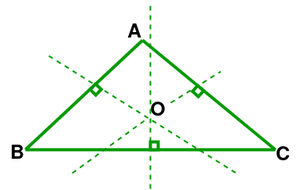To obtain a point which is equidistant from all vertices of a triangle we construct perpendicular bisectors of all sides (AB, BC, CA) of the triangle (ΔABC). The point of intersection of these bisectors is known as Circumcenter(O) which is equidistant from all vertices.

### Question 2. In a triangle locate a point in its interior which is equidistant from all the sides of the triangle.

Solution: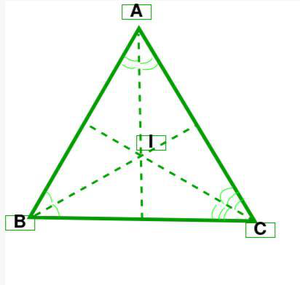To obtain a point which is equidistant from all sides of a triangle we construct angle bisectors of all angles present in ΔABC i.e ∠BAC, ∠ABC, ∠ACB. The point of intersection of these bisectors is called Incentre(I) which is equidistant from all sides.

### (Hint : The parlour should be equidistant from A, B and C)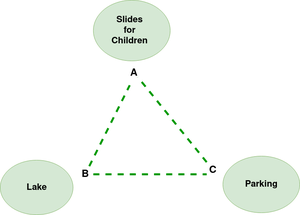Solution: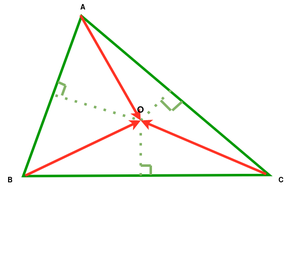The ice-cream parlour must be set somewhere so that it’s easily available for the public. So for such point it should be at a equal distance from point A, B, C & such point is termed as circumcenter.

### Question 4. Complete the hexagonal and star-shaped Rangolies by filling them with as many equilateral triangles of side 1 cm as you can. Count the number of triangles in each case. Which has more triangles?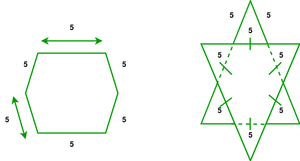Solution: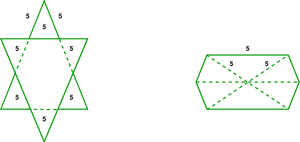We need to find the number of triangles that can get fit the above figures i.e the hexagon and the star.

So,

Area of hexagon = (Area of small triangle inside hexagon) * 6

Area of small equilateral triangle = √3/4 * a

= √3/4 * 52

= √3/4 * 25 = 25√3/4

So,

Area of hexagon = 25√3/4 * 6

= 150√3/4 cm

Area of Star = Area of 6 triangles and 1 hexagon

= 6 * 25√3/4 + 150√3/4

= 300√3/4 cm

Area of triangles of 1cm side that are to be fitted = √3/4 * 12

= √3/4 cm2

Number of triangles that can be accommodated inside hexagon and stars :

a. For Hexagon : Area of hexagon/ Area of 1cm side triangle

= 150√3/4 cm2 / √3/4 cm2

= 150 triangles

b. For Star : Area of star/ Area of 1cm triangle

= 300√3/4 cm2 / √3/4 cm2

= 300 triangles

Hence, the star can accommodate 150 more triangles than the hexagon.

My Personal Notes arrow_drop_up
Related Tutorials